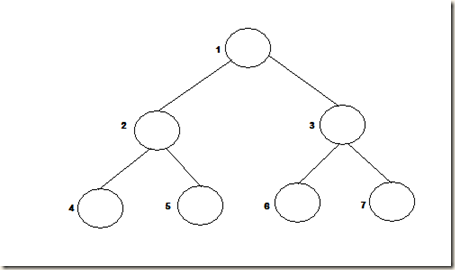Monday, 6 May 2013

Create a binary tree using an array

Introduction

Its a reiterate of the same concept of mapping an array to a binary tree. We use this helper routine for creating binary trees used in our problems. So thought about showcasing the code to do that.

Solution

if you have an array like,

arr[] = { 2,3,6,1,8,2,3}

Total: 7 elements. To form a balanced binary tree out of the above array, we have to follow the below index to node mapping for the tree!!root = i

left = 2i+1

right = 2i+2

(Assuming starting index of array is 0)

For creating the above balanced tree, you need to do a level order traversal.

We already have some examples (See references). But lets think simple way.

Algorithm:

- queue the first node

- loop

- de-queue from the vector, create a new node + left and right with data.

- push left & right of current node into the queue

- exit when array length is exceeded!!

struct BinTree
{
int data;
BinTree *left;
BinTree *right;

BinTree(int data) {
this->data = data;
this->left = NULL;
this->right = NULL;
}
};

BinTree* createBNode(int data)
{
return new BinTree(data);
}

BinTree* arr_to_tree(int *arr, int size)
{
BinTree *newTree, *root;
vector<BinTree*> node_list;
int i=0;
root = createBNode(arr);
node_list.push_back(root);
while(!node_list.empty())
{
newTree = node_list.front();
node_list.erase(node_list.begin());
if(2*i + 1 >= size)
break;

newTree->left = createBNode(arr[2*i+1]);
node_list.push_back(newTree->left);
if(2*i+2 >= size)
break;

newTree->right = createBNode(arr[2*i+2]);
node_list.push_back(newTree->right);
i+=1;
}
return root;
}

void main()
{
BinTree *tree;
int arr[] = { 2,3,4,6};

tree = arr_to_tree(arr,4);

}

Reference